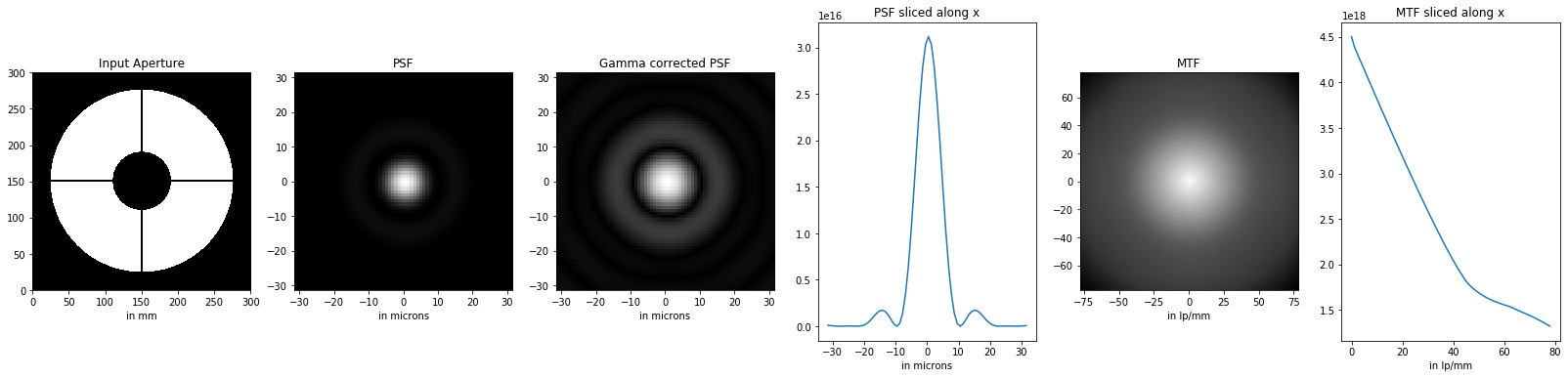# Designing the optimum vanes and spider of a telescope using Fourier Optics

While designing the new spider system for a Newtonian telescope, one question came up: if using a double vane system, what is the optimal spacing between the vanes?

I tried to get the value of the double vane spacing from equivalent commercial telescopes (like the ASA 10 inch) so I could just copy that and move on, but could not find the value. Further, I was uncertain if an optimum value for an f/3 ASA would be optimal for the f/4.5 built considered.

Then I became very curious about that question, and also realized I needed to be able to evaluate rigorously the diffraction pattern created by each one of the spider designs I was considering for the telescope, to make an informed choice for my build.

I kind of knew that to answer the above question, I needed to solve the Fraunhaufer equation over the aperture. A few things (that may seem totally obvious for somebody who has studied Fourier Optics) were quite unclear at that point, and I got some very useful pointers on Cloudy Nights. In the end I got the text book “Fourier Optics” by Goodman, and this is where I should have started, as there is no shortcuts in Physics.

The equation is an integral of complex numbers looking pretty intimidating, however, upon careful examination, it turns out to be the square of a Fourier transform.

This is a well known property, of course, and it has lead to the prolific field of Fourier optics.

So, the numerical integration in Python of the Fraunhofer equation over a given aperture, at a given wavelength and in focus is as simple as this:

``````# im is an image of the aperture (ie 2D numpy array)
f = np.fft.fft2(im)
fshift = np.fft.fftshift(f)

# The amplitude
amp=(np.abs(fshift))

# The Intensity, ie the PSF or Point Spread Function
psf=np.square(amp)

# The MTF or the Modulation Transfer Function
mtf=np.abs(np.fft.fftshift(np.fft.fft2(psf)))``````

That’s it! It just takes one line of code to integrate the Fraunhofer equation! Then one line to get the Point Spread Function and one line to get the Modulation Transfer Function. Lured by this apparent facility, I decided to jump into Fourier Optics and develop my own scrip to evaluate the diffraction pattern of the various aperture obstructions I was envisioning for my project.

After the FFT, PSF and MDF evaluation, there is a second step, converting units from pixels to physical units. This step is often overlooked in textbooks or explanation found on the web, as there is no fundamental difficulty. However it needs to be done correctly and is not that obvious (at least it took me some thinking, and reading this helped). Here is what it looks like in Python:

``````# Your f/ number, a-dimensional.
fn=4.5

# The monochromatic wavelength for the calculation in nanometers
la=656.28

# The spatial scale is Q pixels per mm
Q=4

# Convert la from nano meters to microns and
# Q from pixel/mm to pixel/micron
PSFscale=(la/1000)*fn*(Q/1000) ``````

The rest of the script is a bit of python to glue the pieces together and some matplotlib jargon to get the pictures displayed properly.

The complete script is distributed on my Github, open source.Result of the script: aperture, PSF, PSF corrected with Gamma=0.5, PSF curve along x, MTF, MTF along x.

Some considerations:

• The above integration is valid for one wave length. For the visual impulse response, you would need to further integrate over all the wavelength visible to your eyes.
• This calculation is valid at focus.
• the support array needs to be much bigger than the mask to avoid artefacts in the Fourier transform. This is a numerical calculation trick, but if overlooked, the results are plain wrong. This happened on this forum, and you have to go through pages of posts for somebody to eventually point out the result presented were incorrect in the first place, and suggest the correct solution to solve the problem.
• if new to fft in python/matlab, read and understand fftflip doc.
• with cv2, arrays needs to be normalized before saving to image, otherwise you may end up with an all black image while the pyplot looks fine. I provide such normalization functions in the script, if you want to save directly to .png.

Other resources worth checking: http://aberrator.astronomy.net/.

So, after disappearing in this rabbit hole for a long time, what is the optimum spacing for a double vane system? Well, it does not matter. I will write on this more at some point.

Another way to put it is: the optimal spacing of the vanes is dictated by mechanical constraints, not optical constraints.